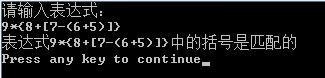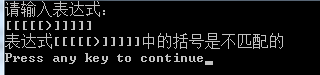• 顺序栈判断表达式括号是否匹配
顺序栈判断表达式的括号匹配
代码如下：
#include <iostream>
using namespace std;
typedef struct sqstack                   //顺序栈定义
{
char data;
int top;
}Sqstack;

void initstack(struct sqstack &Sqstack) //初始化栈
{
Sqstack.top=-1;
}

int isempty(struct sqstack &Sqstack)    //判断栈是否空
{
if(Sqstack.top==-1)
{
cout<<" 顺序栈为空 "<<endl;
return 1;
}
else
{
cout<<" 顺序栈不为空 "<<endl;
}
return 0;
}

int push(struct sqstack &Sqstack,char x)  //插入元素x作为新的栈顶元素
{
if(Sqstack.top==9)
{
cout<<" 错误 "<<endl;
return 0;
}
Sqstack.top=Sqstack.top+1;
Sqstack.data[Sqstack.top]=x;
return 1;
}

int gettop(struct sqstack Sqstack,char &e)//得到栈顶元素的值
{
if(Sqstack.top==-1)
{
cout<<" NULL(空表) "<<endl;
return 0;
}

e=Sqstack.data[Sqstack.top];
return 1;

}
int pop(struct sqstack &Sqstack,char &x)//删除栈顶元素并用x返回它
{
if(Sqstack.top==-1)
{
return 0;
}
x=Sqstack.data[Sqstack.top];
Sqstack.top=Sqstack.top-1;
return 1;
}

int ifmatch(char exp[],int n)//从字符串中读取元素，
//若为左括号则将它压进栈中再读取到右括号则将出栈（消除一对括号），重复此步骤直到读完字符串。若栈为空说明一对对括号消除即括号匹配，若不为空说明右括号比左括号多即不匹配
//若为右括号则直接说明不匹配
{
struct sqstack stack;//结构体栈创建实例
initstack(stack);//初始化
int i;int x;
for(i=0;i<n;i++)
{
if(exp[i]=='(')
{push(stack,'(');}
if(exp[i]==')')
{
if(isempty==1)//栈空且只有右括号说明不匹配
{return 0;}
else
{pop(stack,x);}
}
}
if(isempty==1)//栈空说明括号一对对消除则匹配
{return 1;}
else
{return 0;}//栈不空说明站内还剩下未消除的左括号则不匹配
}

int main()
{
char exp;
int i=0;
cout<<"请输入表达式：";
cin>>exp;
while(exp[i]!='\0')//求输入的字符串长度
{i++;}
if(ifmatch(exp,i)==1)
{cout<<"匹配";}
else
{cout<<"不匹配";}
return 0;
}

运行结果如下：展开全文数据结构 c++
• js关于判断算式中括号匹配的问题 【js数据结构】解决括号匹配问题
js关于判断算式中括号匹配的问题
【js数据结构】栈解决括号不匹配问题


展开全文• 《数据结构，算法及应用》 zhangxianchao P80 13题
• 题目：设计一个算法，判断用户输入的表达式括号是否匹配表达式中可能含有圆括号、中括号和大括号。思路：建立一个顺序，当表达式中有左括号时将其入栈，当出现右括号时，将栈顶元素出栈，检查与当前右括号是否...
题目：设计一个算法，判断用户输入的表达式中括号是否匹配，表达式中可能含有圆括号、中括号和大括号。

思路：建立一个顺序栈，当表达式中有左括号时将其入栈，当出现右括号时，将栈顶元素出栈，检查与当前右括号是否匹配。最后如果栈为空则表示该表达式中的括号是匹配的。

代码：

#include<iostream>
#include<string>
using namespace std;
#define MaxSize 20
//字符串栈
class Stack
{
char *data;
int top;
public:
Stack();
~Stack();
bool IsEmpty();
bool Push(char e);
bool Pop(char& e);
};

Stack::Stack()
{
data = new char[MaxSize];
top = -1;
}

Stack::~Stack()
{
delete [] data;
}

bool Stack::IsEmpty()
{
return (top == -1);
}

bool Stack::Push(char e)
{
if(top == MaxSize-1)    return false;   //栈满
top++;
data[top] = e;
return true;
}

bool Stack::Pop(char& e)
{
if(top == -1)   return false;   //栈空
e = data[top];
top--;
return true;
}

bool IsMatch(char str[],int n)
{
int i=0;
char e;
Stack st;   //建立顺序栈
while(i<n)
{
if(str[i] == '(' || str[i] == '[' || str[i] == '{')
st.Push(str[i]);
else
{
if(str[i] == ')')
{
if(!st.Pop(e))  return false;
if(e!='(')  return false;
}
if(str[i] == ']')
{
if(!st.Pop(e))  return false;
if(e!='[')  return false;
}
if(str[i] == '}')
{
if(!st.Pop(e))  return false;
if(e!='{')  return false;
}
}
i++;
}
if(st.IsEmpty())    return true;
else return false;  //遍历字符串后栈不为空说明有不匹配字符
}

void main()
{
cout<<"请输入表达式："<<endl;
char str[MaxSize];
cin>>str;
int n = strlen(str);
if(IsMatch(str,n))
cout<<"表达式"<<str<<"中的括号是匹配的"<<endl;
else
cout<<"表达式"<<str<<"中的括号是不匹配的"<<endl;
}

测试数据1：9*{8+[7-(6+5)]}
测试结果1：测试数据2：[[[[[)]]]]]
测试结果2：展开全文数据结构 算法 c++
• 昨天在群里有个同学问怎么校验括号是否匹配， 首先想到的是，遍历字符，如果遇到(,[,{就入栈，如果遇到),],}就弹出。很快代码如下实现了，这里要说的是java集合中有个这个数据结构，不需要自己再实现一个，...

昨天在群里有个同学问怎么校验括号是否匹配，
首先想到的是栈，遍历字符，如果遇到(,[,{就入栈，如果遇到),],}就弹出栈。很快代码如下实现了，这里要说的是java集合中有个栈这个数据结构，不需要自己再实现一个，所以比较快。代码如下：
<strong style="padding: 0px; margin: 0px;"><code style="padding: 0px; margin: 0px;">package pipei;
import java.util.Stack;
/***
*
*【描述】：括号匹配
*【作者】：alaric
*【时间 】：Jun 4, 2012
*【文件】：pipeiMatching.java
*
*/
public class Matching {
public static boolean Match(String str){
char [] charArray = str.toCharArray();
Stack stack = new Stack();
for(char c: charArray){
if(c==’{‘||c==’['||c=='('){
stack.push(c);
}
if(c==')'||c==']‘||c==’}'){
Character cc= stack.pop();
switch(c){
case ‘)’: if(cc!=’(‘)return false;break;
case ‘]’: if(cc!=’[')return false;break;
case '}': if(cc!='{')return false;break;
}
}
}
return true;
}
/**
*【描述】：括号匹配测试
*【作者】：alaric
*【时间 】：Jun 4, 2012
*
*/
public static void main(String[] args) {
// TODO Auto-generated method stub
String string=”[[(()eeee)]]”;
if (Match(string)) {
System.out.println(“匹配”);
}else {
System.out.println(“不匹配”);
}
}
}</code></strong>

当我发出代码的时候，他说他要js写的，java看不懂，我崩溃，js实现个栈还是需要点时间的，于是拖到今天，终于有空实现下了，
代码如下：

<!DOCTYPE html PUBLIC “-//W3C//DTD HTML 4.01 Transitional//EN” “http://www.w3.org/TR/html4/loose.dtd”>
<html>
<head>
<meta http-equiv=”Content-Type” content=”text/html; charset=UTF-8″>
<title>Insert title here</title>
</head>
<body>
<script>
function Stock(count)
{
this.ptCount=-1;//指向最后一个数据的指针
this.count=100;//容量
this.myArray=new Array();
if(count!=undefined)
{
this.count=count;
this.myArray=new Array(this.count);
}else{
this.count=0;
}
this.Info=function(mvalue)//进栈
{
if (this.ptCount == this.count)
{
return false;
}else{
++this.ptCount;
this.myArray[this.ptCount] = mvalue;
return true;
}
return false;
}
this.Out=function()//出栈
{
if (this.ptCount == -1)
{
return false;
}
else
{
var reObject;
reObject = this.myArray[this.ptCount];
this.ptCount–;
return reObject;
}
}
this.RLCount=function()
{
return this.ptCount + 1;
}
this.Clear=function()
{
this.ptCount=-1;
}
}
function Match(str){
var charArray =new Array();
charArray= str.split(“”);
var stack = new Stock(100);
for(var i=0;i<charArray.length;i++){
var c= charArray[i];
if(c==’{‘||c==’['||c=='('){
stack.Info(c);
}
if(c==')'||c==']‘||c==’}'){
var cc= stack.Out();
switch(c){
case ‘)’: if(cc!=’(‘)return false;break;
case ‘]’: if(cc!=’[‘)return false;break;
case ‘}’: if(cc!=’{‘)return false;break;
}
}
}
if(stack.Out())return false;
return true;
}
function test(){
var str = document.getElementById(“strId”).value
var b = Match(str);
document.getElementById ( “outId” ).value=b;
}
</script>
<input type=”text” id=”strId” value=”"/>
<input type=”text” id=”outId” value=”"/>
<input type=”button” οnclick=”test();” value=”submit” />
</body>
</html>

js 栈的实现，稍微有点麻烦，至少你要明白什么是栈这种数据结构，如果你了解了，这里这个代码不是很困难的。

展开全文•数据结构 算法
• 建立一个，顺序对表达式进行扫描，如遇到‘（’，‘【’，‘{’，左半边括号将其入栈，遇到右半边括号，检查当前栈顶元素是否对应，对应就出栈，不对应则不匹配。 Ps：主要注意出栈与入栈对栈顶的操作的先后...数据结构
• //判断一个算术表达式的圆括号是否正确配对 #include #define MAXSIZE 1000 typedef char datatype; typedef struct Stack{ datatype elements[MAXSIZE]; int Top; }stacky; stacky *SetnullS(stacky *S){ S->...c
• 请实现一个算法用于消除表达式内嵌套的括号，例如将上面的表达式变为(a,b,1,2,c,a,5)，如果表达式有误请报错。 思路： 利用的特点，遇到 左括号 ( ，则入栈； 遇到右括号 ) ，则出栈。注意出栈时检查栈是否为空...
•  *判断表达式左右括号是否匹配  *把表达式中的字符按照顺序读取，碰到左括号就压入stack中，碰到右括号就  *先判断stack是否为空，不为空就把stack中的顶层弹出，看是否和右括号匹配。  *最后根据stack中应为空...string class
• 试编写一个函数，判断表达式中的括号是否匹配？ 方法一：利用 方法二：首尾各一个指针，在向中间移动的过程中判断 方法三：递归 不好理解的递归实现： #include #include #define FAIL -1 #define SUCCESS 0 ...
• 设计一个算法判断表达式中的括号是否匹配 二、问题解答 解析：这里需要用到STL在算法设计中的应用，STL在算法设计中的应用有如下几种： Ⅰ.存放主数据 ⭐ Ⅱ.存放临时数据 Ⅲ.检测数据元素的唯一性 Ⅳ.数据的排序 Ⅴ...STL 堆栈 后进先出
• ps: 判断一个数学表达式括号是否匹配，不仅要判断其左右大小括号的数量是否对应相等，还要判断括号的匹配次序是否正确。 算法思想: 顺序扫描算数表达式（表现为一个字符串），当遇到三种类型的左括号时候，执行...数据结构 算法
• 设计一个算法采用顺序栈判断表达式中的括号是否正确匹配。 解题 基本思路如下：实例化一个字符栈，维持一个bool变量flag，初始化为true。然后遍历整个表达式： 如果是三种正括号‘(’ '[' '{'，则入栈； ...C++
• 是一种后入先出（LIFO，last-in-first-out）的数据结构。 假设有一个算术表达式 ...利用后入先出的这个特点，对字符串（算术表达式）进行遍历，如果遇到左括号"("，就把字符"("压入栈 for (let i = 0; i ...
• 可以用来判断一个算术表达式中的括号是否匹配。编写一个函数，该函数接受一个算术表达式作为参数，返回括号缺失的位置。下面是一个括号不匹配的算术表达式的例子：2.3 + 23 / 12 + (3.14159×0.24。 首先，我们都...数据结构
• 元旦三天假，闲着没事干，就想着复习一下学数据结构时的那些算法吧。本来是想用C语言来写的，无奈啊，三四年没用C了，基本上忘光光，还是用C#来写吧，而且.Net基类库中已经有了... 对于表达式中的括号是否匹配，不能...
• 利用栈判断输入的表达式中的括号是否匹配（假设只含有左、右括号） bool Match(char exp[],int n) {  int i=0;  char e;  bool match=true;  SqStack *st;  InitStack(st);//初始化栈   while(i ...数据结构
• （洛谷P1739 表达式括号匹配） 题目 见题目链接 题目链接表达式括号匹配 - 洛谷 输入 见题目链接 输出 见题目链接 样例 见题目链接 题解 用一个来存储做括号，读取输入的时候，每读到一个左括号则压入栈，碰到右...数据结构
• 判断一个表达式的左右括号是否匹配 int IfMatch(char s[]){ int top=-1,i=0; while(s[i]){ if(s[i]=='(') top++; else if(s[i]==')'){ if(top!=-1) top--; else return 0;//右括号多，（...c语言
• 我们都知道，（stack）具有后进先出的特点，所以在我们思考一个表达式中的左右括号是否匹配问题时，就自然会想到是不是可以利用的特点来判断左右括号是否匹配呢？ 主要思路如下： 1.扫描整个表达式； 2.判断...
• 数据结构上机作业。数据结构张宪超第二章13题。 假设表达式中允许包含3种括号：圆括号，方括号，大括号。设计算法采用顺序栈判断表达式中的括号是否正确配对。
• 题目大意： 给定一个字符串，判断其中的左右括号是否配对 题目分析： 洛谷归类在线性结构里面，应该是用，那么我们遇到左括号，就在里面放一个1，遇到右括号就取出一个1来，如果结束的时候还有1在里面，就是......